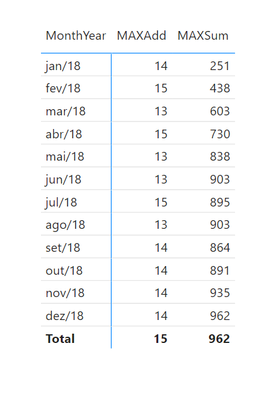cancel
Showing results for
Did you mean:Resolver I

Hey guys,

I've a huge dataset and I must summarize some of its values into a calendar-like table in order to better perform calculations with my measures. In this file I've created a smaller one just to exemplify what I aim to do.

The table that's generated off the SUMMARIZE measure brings the correct result, as follows:

``````@Summarized_tbl =
SUMMARIZE(F_Table,
d_Calendario[Date],
"@count",	VAR MAXDATE = MAX(d_Calendario[Date])
VAR MINDATE = MIN(d_Calendario[Date])
VAR CALCULO = COUNTROWS(FILTER(ALL(F_Table),
[start]	<= 	MAXDATE	&&
[end]	>=	MINDATE))
RETURN CALCULO)``````

Date  |   @count

 1/1/2018 5 1/2/2018 14 1/3/2018 19 1/4/2018 30 1/5/2018 37 1/6/2018 43 1/7/2018 51 1/8/2018 58 1/9/2018 67 1/10/2018 73 1/11/2018 84 1/12/2018 93 1/13/2018 96 1/14/2018 99 1/15/2018 108

However, in my real dataset I'm not able to build this table using SUMMARIZE due to performance issues (whenever I need to create a column or a measure it takes almost two minutes), so that's the reason why I'm trying to create this table using ADDCOLUMNS, which in turn brings the wrong result, as follows:

``````@AddC_tbl =
VALUES(d_Calendario[Date]),
"@count",	VAR MAXDATE = MAX(d_Calendario[Date])
VAR MINDATE = MIN(d_Calendario[Date])
VAR CALCULO = CALCULATE(
COUNTROWS(
FILTER(F_Table,
[start]	<= 	MAXDATE	&&
[end]	>=	MINDATE)))
RETURN CALCULO)``````

Date  |   @count

 01/01/2018 5 02/01/2018 9 03/01/2018 6 04/01/2018 11 05/01/2018 7 06/01/2018 7 07/01/2018 9 08/01/2018 7 09/01/2018 9 10/01/2018 6 11/01/2018 11 12/01/2018 9 13/01/2018 3 14/01/2018 3 15/01/2018 9

This calculation is simply counting how many records started on the row context date, but what I want to do is to count how many records was active on each month, as follows:Any thoughts on what I'm doing wrong about tha ADDCOLUMNS DAX measure previously shown?

1 ACCEPTED SOLUTIONResolver I

The solution was wrapping the MAX and MIN formulas into CALCULATE and on the filter inside the COUNTROWS put an ALL.

``````@AddC_tbl =
VALUES(d_Calendario[Date]),
"@count",	VAR MAXDATE = CALCULATE(MAX(d_Calendario[Date]))
VAR MINDATE = CALCULATE(MIN(d_Calendario[Date]))
VAR CALCULO = CALCULATE(
COUNTROWS(
FILTER(ALL(F_Table),
[start]	<= 	MAXDATE	&&
[end]	>=	MINDATE)))
RETURN CALCULO)``````

3 REPLIES 3Resident Rockstar

HI @Pedro503 ,

Can you maybe show the data model and sample data?

Because if I do not miss something then the following measure should bring the same result like the first measure and if it does then it also should be more performant.

``````@Summarized_tbl =

SUMMARIZE(
d_Calendario,
d_Calendario[Date],
"@count",
COUNTROWS(F_Table)
)``````

I come to this conclusion because you calculate a min and max on the same column which you use for your summarization and then you do another calculation using the min and max. Fomr my understanding you have a date table and a fact table with 1:n relation ship and you want the count of rows per date. If that is the case the measure above should do the job as well as should be more performant.

Or am I missing here something?

Best regards

Michael

-----------------------------------------------------

If this post helps, then please consider Accept it as the solution to help the other members find it more quickly. Appreciate your thumbs up!

-----------------------------------------------------

------------------------------------------------------------------
Visit my blog datenhungrig which I recently started with content about business intelligence and Power BI in German and English or follow me on LinkedIn!Resolver I

The solution was wrapping the MAX and MIN formulas into CALCULATE and on the filter inside the COUNTROWS put an ALL.

``````@AddC_tbl =
VALUES(d_Calendario[Date]),
"@count",	VAR MAXDATE = CALCULATE(MAX(d_Calendario[Date]))
VAR MINDATE = CALCULATE(MIN(d_Calendario[Date]))
VAR CALCULO = CALCULATE(
COUNTROWS(
FILTER(ALL(F_Table),
[start]	<= 	MAXDATE	&&
[end]	>=	MINDATE)))
RETURN CALCULO)``````Resolver I

Dont know what's happening, but I cant paste the link here (the second line contains the link). The file is on the second line. Actually what I aim to do is to count how many records exists on every month, not necessarily to just count the rows.

For instance, some Id started on 01/01/2018 and was active untill 10/01/2018. I wanna indicate that on every month from January to October this subscription was active.

 Jan 1 Feb 1 Mar 1 Apr 1 May 1 Jun 1 Jul 1 Aug 1 Sep 1 Oct 1

To do so, I compute the following Dax measure:

``````COUNTROWS(
FILTER(ALL(F_Table),

[start] <= MAXDATE &&

[end]   >= MINDATE))``````

Do you know how to enhance its performance using ADDCOLUMNS?Announcements#### Exclusive opportunity for Women!

Join us for a free, hands-on Microsoft workshop led by women trainers for women where you will learn how to build a Dashboard in a Day!#### Power Platform Conference-Power BI and Fabric Sessions

Join us Oct 1 - 6 in Las Vegas for the Microsoft Power Platform Conference.Top Solution Authors
Top Kudoed Authors
Users online (1,589)